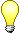Untitled Document

This is schematic of a synthesized PLL for the Low Power FM transmitter. By the way, you also can use it with other circuits, just pay attention to recalculate the loop filter response and components, VCO tank circuit, and the correct thumbswitch programming keys, crystal value.

The original project of FM transmitter was made to operate in both configuration ( synthesized or open loop free oscilattor), with synthesized version you can assure very high frequency stability, and also lock digital FM receivers ( 200KHz step in major of countries).

A crystal reference of 5.12 MHz was employed to assure 10 KHz steps ( enough sufficient to FM channeling of 200 KHz), and it also depends of R divider crystal reference, here I used R=512 to the MC145151P2 of Motorola.

Since you are using a single modulus PLL IC, the formula to find the required step frequency is :

Fxtal/R = Fvco/N*P (for lock frequency condition)

Fxtal - is the fundamental ressonance crystal frequency.

R - is the internal reference divider of PLL ( to MC145151P2 it can assume a range of 8 to 8192)

Fvco - is the output frequency of your Voltage Control Oscilattor

N - is the internal programming frequency divider ( base 2 , N9 to N0 for the MC145151P2)

P - is the prescaler divider, normally P=40 to MC12016, the PLL needs downconverter the input frequency ( pin1) because the phase/frequency comparator just work at maximum of 10MHz or 15MHz input frequency.

click here to view schematic